# Stochastic Parameters¶

## Introduction¶

When augmenting images during experiments, usually one wants to augment each image in different ways. E.g. when rotating images, not every image is supposed to be rotated by 10 degrees. Instead, only some are supposed to be rotated by 10 degrees, while others should be rotated by 17 degrees or 5 degrees or -12 degrees - and so on. This can be achieved using random functions, but reimplementing these, making sure that they generate the expected values and getting them to work with determinism is cumbersome. To avoid all of this work, the library uses Stochastic Parameters. These are usually abstract representations of probability distributions, e.g. the normal distribution N(0, 1.0) or the uniform range [0.0, 10.0]. Basically all augmenters accept these stochastic parameters, making it easy to control value ranges. They are all adapted to work with determinism out of the box.

The below code shows their usage:

```from imgaug import augmenters as iaa
from imgaug import parameters as iap

seq = iaa.Sequential([
iaa.GaussianBlur(
sigma=iap.Uniform(0.0, 1.0)
),
iaa.ContrastNormalization(
iap.Choice(
[1.0, 1.5, 3.0],
p=[0.5, 0.3, 0.2]
)
),
iaa.Affine(
rotate=iap.Normal(0.0, 30),
translate_px=iap.RandomSign(iap.Poisson(3))
),
iap.Discretize(
(iap.Beta(0.5, 0.5) * 2 - 1.0) * 64
)
),
iaa.Multiply(
iap.Positive(iap.Normal(0.0, 0.1)) + 1.0
)
])
```
The example does the following:
• Blur each image by sigma, where sigma is sampled from the uniform range [0.0, 1.0). Example values: 0.053, 0.414, 0.389, 0.277, 0.981.
• Increase the contrast either to 100% (50% chance of being chosen) or by 150% (30% chance of being chosen) or 300% (20% chance of being chosen).
• Rotate each image by a random amount of degrees, where the degree is sampled from the normal distribution N(0, 30). Most of the values will be in the range -60 to 60.
• Translate each image by n pixels, where n is sampled from a poisson distribution with alpha=3 (pick should be around x=3). As we cant translate by a fraction of a pixel, we pick a discrete distribution here, which poisson is. However, we do not just want to translate towards the right/top (only positive values). So we randomly flip the sign sometimes to get negative pixel amounts too.
• Add to each pixel a random value, sampled from the beta distribution Beta(0.5, 0.5). This distribution has its peaks around 0.0 and 1.0. We multiply this with 2 and subtract 1 to get it into the range [-1, 1]. Then we multiply by 64 to get the range [-64, 64]. As we beta distribution is continuous, we convert it to a discrete distribution. The result is that a lot of pixel intensities are shifted by -64 or 64 (or a value very close to these two). Some other pixel intensities are kept (mostly) at their old values.
• We use Multiply to make each image brighter. The brightness increase is sampled from a normal distribution, converted to have only positive values. So most values are expected to be in the range 0.0 to 0.2. We add 1.0 to set the brightness to 1.0 (100%) to 1.2 (120%).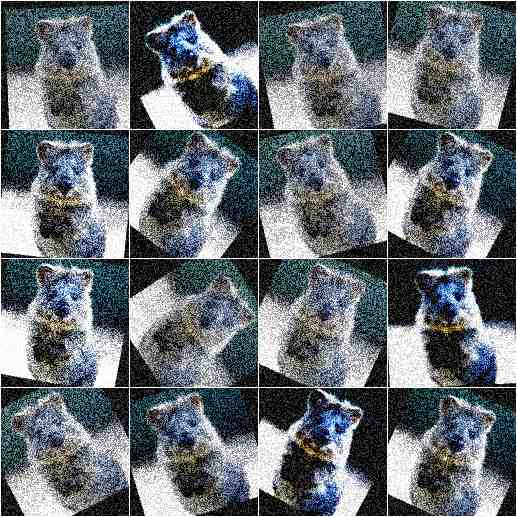## Continuous Probability Distributions¶

The following continuous probability distributions are available:

• Normal(loc, scale): The popular normal distribution with mean loc and standard deviation scale. Example:

```from imgaug import parameters as iap
params = [
iap.Normal(0, 1),
iap.Normal(5, 3),
iap.Normal(iap.Choice([-3, 3]), 1),
iap.Normal(iap.Uniform(-3, 3), 1)
]
iap.show_distributions_grid(params)
```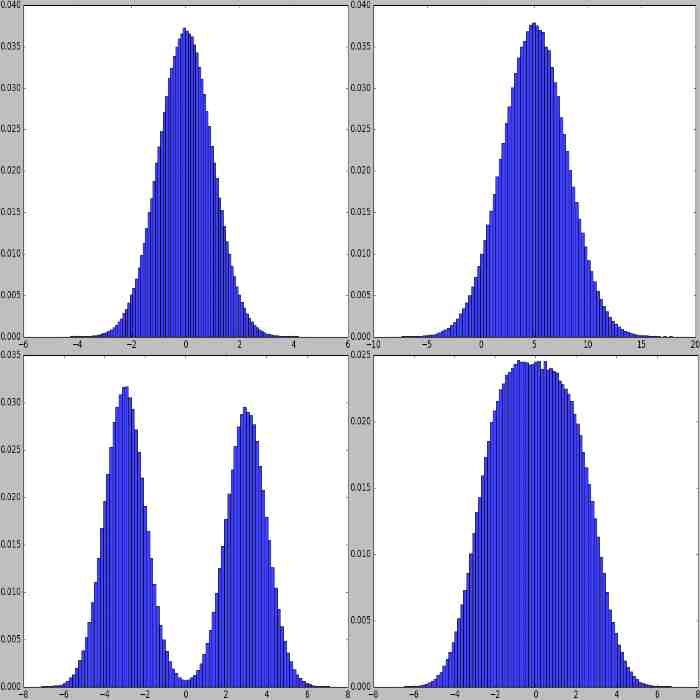• Laplace(loc, scale): Similarly shaped to a normal distribution. Has its peak at loc and width scale. Example:

```from imgaug import parameters as iap
params = [
iap.Laplace(0, 1),
iap.Laplace(5, 3),
iap.Laplace(iap.Choice([-3, 3]), 1),
iap.Laplace(iap.Uniform(-3, 3), 1)
]
iap.show_distributions_grid(params)
```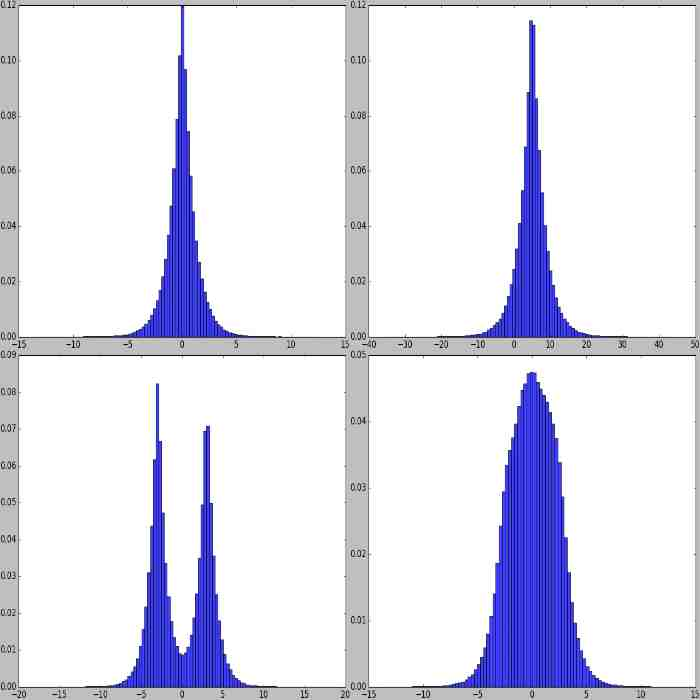• ChiSquare(df): The chi-square (“X^2”) distribution with df degrees of freedom.

Roughly similar to a continuous version of the poisson distribution. Has its peak at df and no negative values, only positive ones. Example:

```from imgaug import parameters as iap
params = [
iap.ChiSquare(1),
iap.ChiSquare(3),
iap.ChiSquare(iap.Choice([1, 5])),
iap.RandomSign(iap.ChiSquare(3))
]
iap.show_distributions_grid(params)
```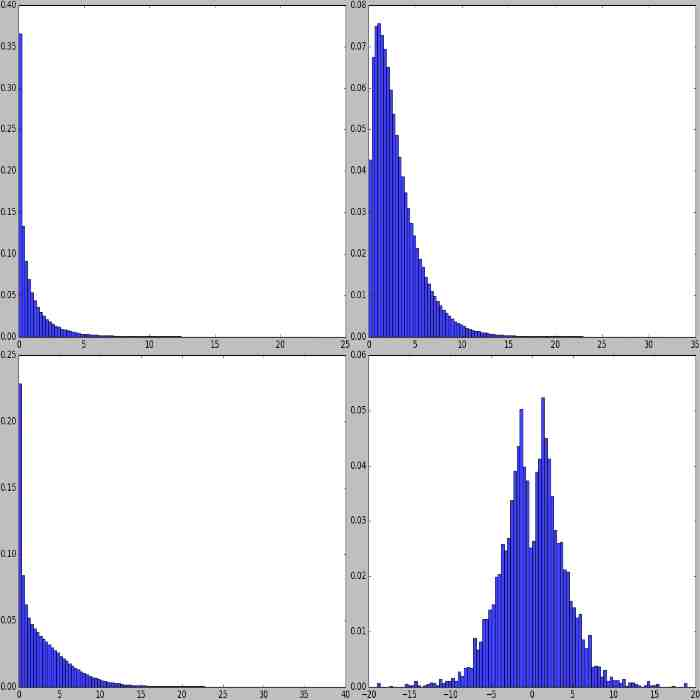• Weibull(a): Weibull distribution with shape a. Example:

```from imgaug import parameters as iap
params = [
iap.Weibull(0.5),
iap.Weibull(1),
iap.Weibull(1.5),
iap.Weibull((0.5, 1.5))
]
iap.show_distributions_grid(params)
```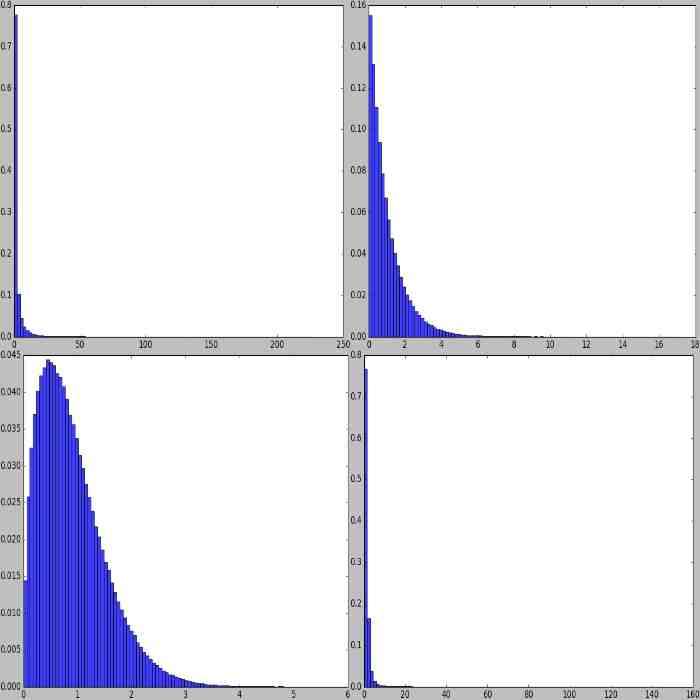• Uniform(a, b): Uniform distribution in the range [a, b). Example:

```from imgaug import parameters as iap
params = [
iap.Uniform(0, 1),
iap.Uniform(iap.Normal(-3, 1), iap.Normal(3, 1)),
iap.Uniform([-1, 0], 1),
iap.Uniform((-1, 0), 1)
]
iap.show_distributions_grid(params)
```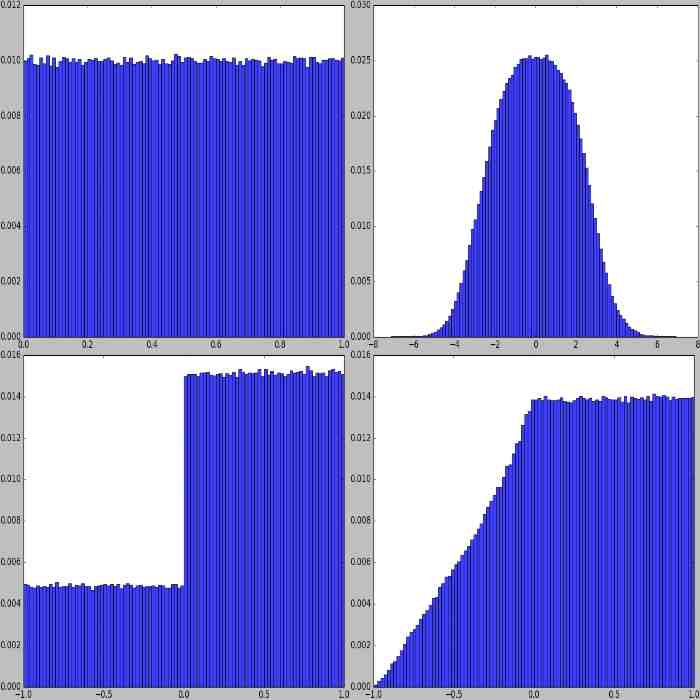• Beta(alpha, beta): Beta distribution with parameters alpha and beta. Example:

```from imgaug import parameters as iap
params = [
iap.Beta(0.5, 0.5),
iap.Beta(2.0, 2.0),
iap.Beta(1.0, 0.5),
iap.Beta(0.5, 1.0)
]
iap.show_distributions_grid(params)
```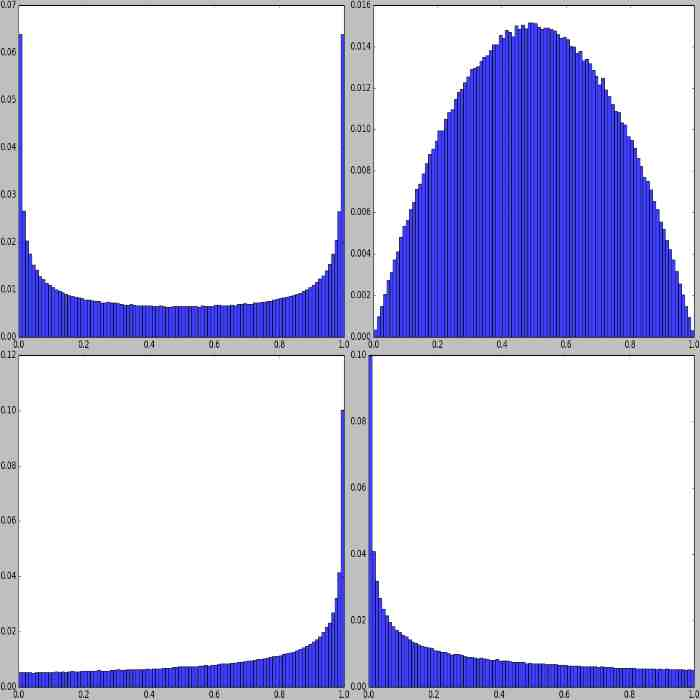## Discrete Probability Distributions¶

The following discrete probability distributions are available:

• Binomial(p): The common binomial distribution with probability p. Useful to simulate coinflips. Example:

```from imgaug import parameters as iap
params = [
iap.Binomial(0.5),
iap.Binomial(0.9)
]
iap.show_distributions_grid(params)
```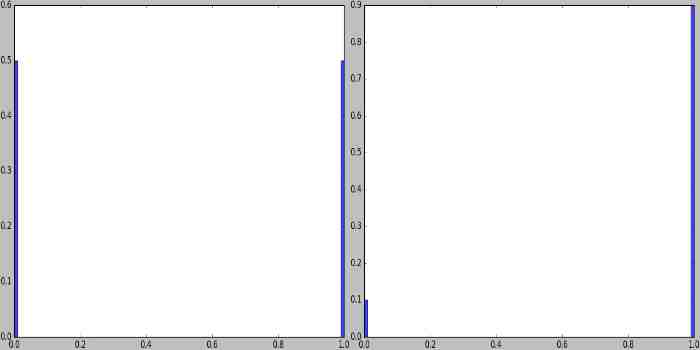• DiscreteUniform(a, b): The discrete uniform distribution in the range [a..b]. Example:

```from imgaug import parameters as iap
params = [
iap.DiscreteUniform(0, 10),
iap.DiscreteUniform(-10, 10),
iap.DiscreteUniform([-10, -9, -8, -7], 10),
iap.DiscreteUniform((-10, -7), 10)
]
iap.show_distributions_grid(params)
```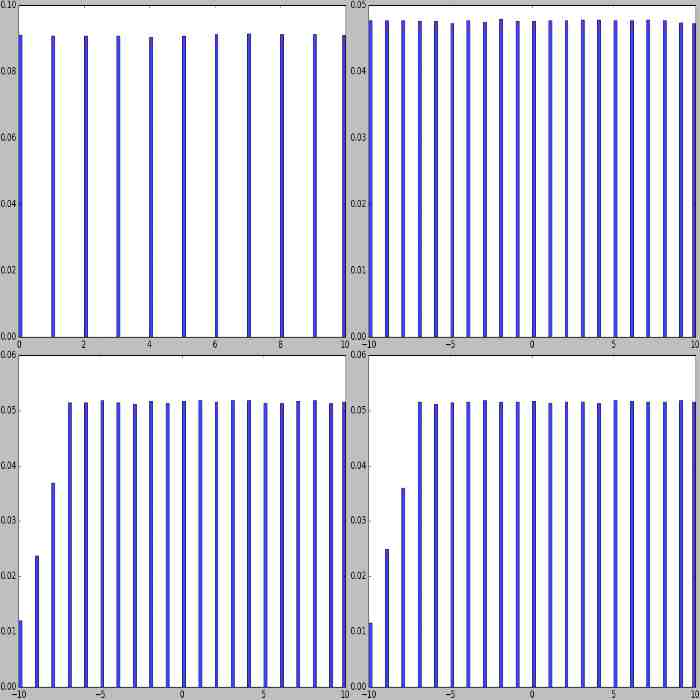• Poisson(lam): Poisson distribution with shape lam. Generates no negative values. Example:

```from imgaug import parameters as iap
params = [
iap.Poisson(1),
iap.Poisson(2.5),
iap.Poisson((1, 2.5)),
iap.RandomSign(iap.Poisson(2.5))
]
iap.show_distributions_grid(params)
```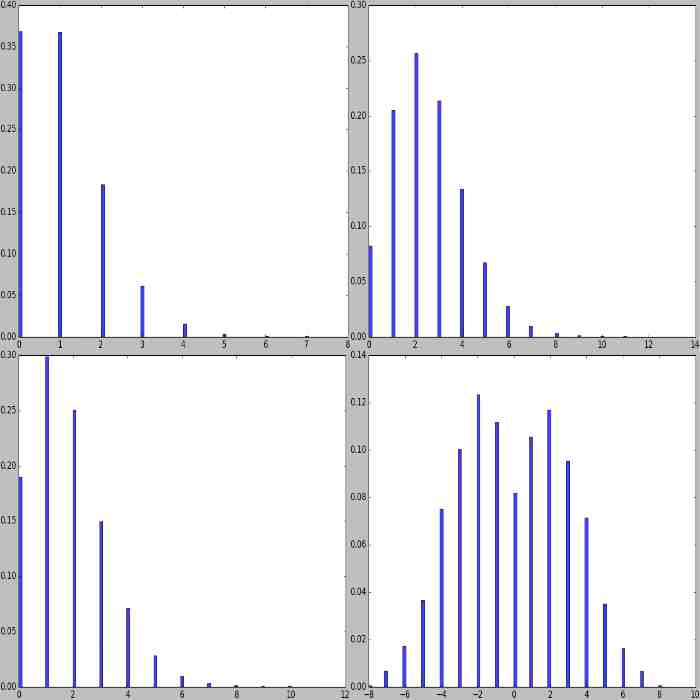## Arithmetic¶

The library supports arithmetic operations on stochastic parameters. This allows to modify values sampled from distributions or combine several distributions with each other.

• Add(param, val, elementwise): Add val to the values sampled from param. The shortcut is +, e.g. Uniform(…) + 1. val can be a stochastic parameter itself. Usually, only one value is sampled from val per sampling run and added to all samples generated by param. Alternatively, elementwise can be set to True in order to generate as many samples from val as from param and add them elementwise. Note that Add merely adds to the results of param and does not combine probability density functions (see e.g. example image 3 and 4). Example:

```from imgaug import parameters as iap
params = [
iap.Uniform(0, 1) + 1, # identical to: Add(Uniform(0, 1), 1)
iap.Add(iap.Uniform(0, 1), iap.Choice([0, 1], p=[0.7, 0.3])),
iap.Normal(0, 1) + iap.Uniform(-5.5, -5) + iap.Uniform(5, 5.5),
iap.Normal(0, 1) + iap.Uniform(-7, 5) + iap.Poisson(3),
]
iap.show_distributions_grid(
params,
rows=2,
sample_sizes=[ # (iterations, samples per iteration)
(1000, 1000), (1000, 1000), (1000, 1000),
(1000, 1000), (1, 100000), (1, 100000)
]
)
```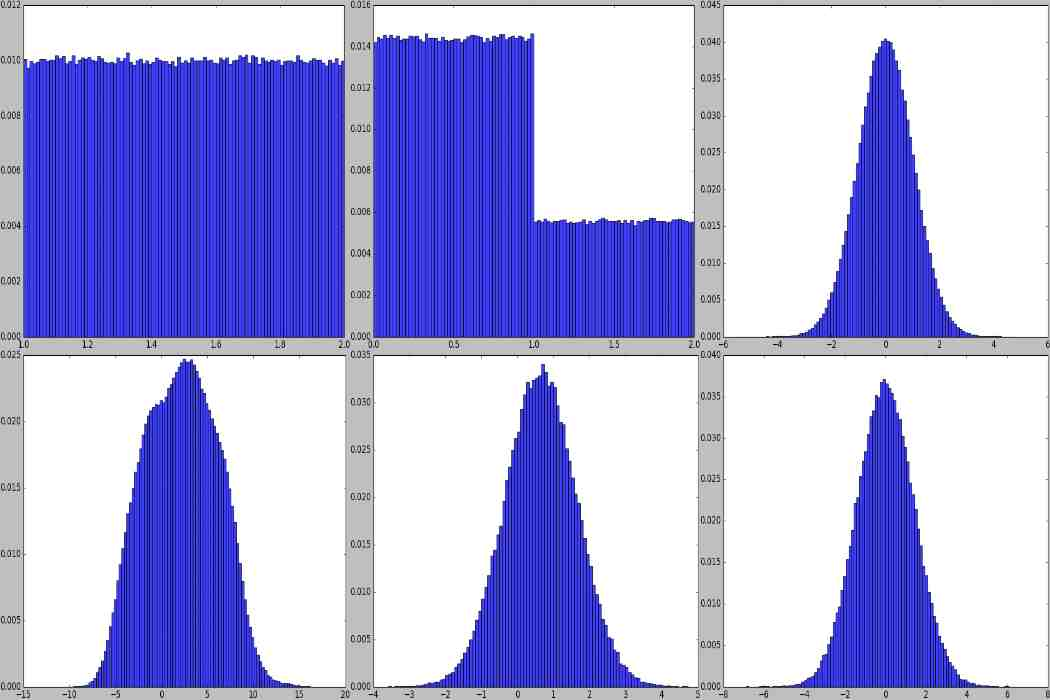• Subtract(param, val, elementwise): Same as Add, but subtracts val from the results of param. The shortcut is -, e.g. Uniform(…) - 1.

• Multiply(param, val, elementwise): Same as Add, but multiplies val with the results of param. The shortcut is *, e.g. Uniform(…) * 2. Example:

```from imgaug import parameters as iap
params = [
iap.Uniform(0, 1) * 2, # identical to: Multiply(Uniform(0, 1), 2)
iap.Multiply(iap.Uniform(0, 1), iap.Choice([0, 1], p=[0.7, 0.3])),
(iap.Normal(0, 1) * iap.Uniform(-5.5, -5)) * iap.Uniform(5, 5.5),
(iap.Normal(0, 1) * iap.Uniform(-7, 5)) * iap.Poisson(3),
iap.Multiply(iap.Normal(-3, 1), iap.Normal(3, 1)),
iap.Multiply(iap.Normal(-3, 1), iap.Normal(3, 1), elementwise=True)
]
iap.show_distributions_grid(
params,
rows=2,
sample_sizes=[ # (iterations, samples per iteration)
(1000, 1000), (1000, 1000), (1000, 1000),
(1000, 1000), (1, 100000), (1, 100000)
]
)
```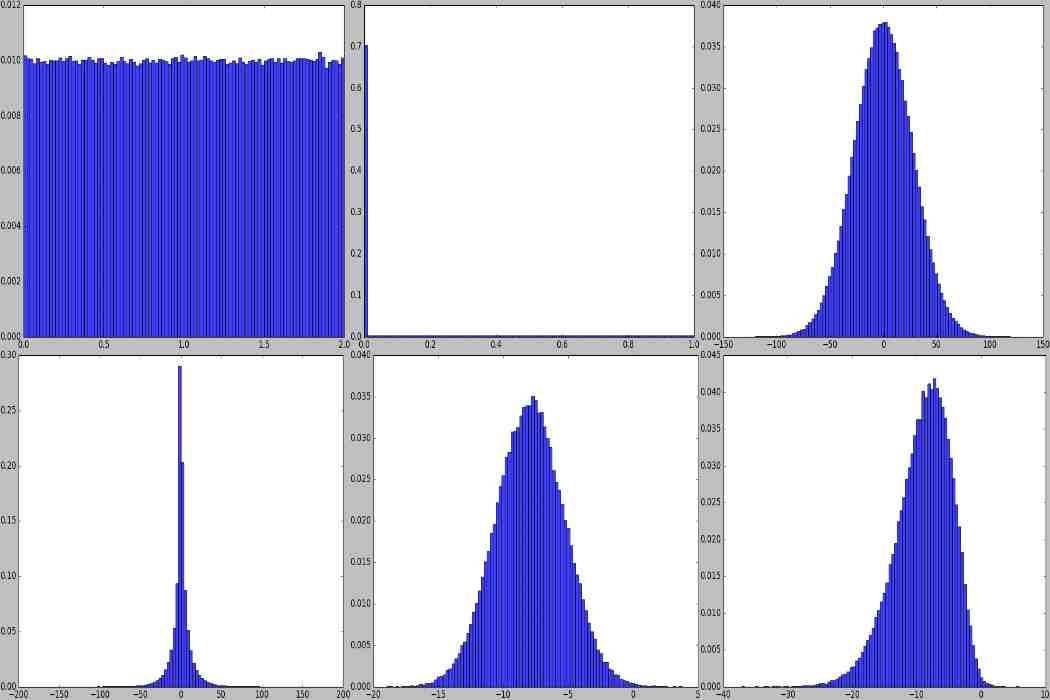• Divide(param, val, elementwise): Same as Multiply, but divides by val. The shortcut is /, e.g. Uniform(…) / 2. Division by zero is automatically prevented (zeros are replaced by ones). Example:

```from imgaug import parameters as iap
params = [
iap.Uniform(0, 1) / 2, # identical to: Divide(Uniform(0, 1), 2)
iap.Divide(iap.Uniform(0, 1), iap.Choice([0, 2], p=[0.7, 0.3])),
(iap.Normal(0, 1) / iap.Uniform(-5.5, -5)) / iap.Uniform(5, 5.5),
(iap.Normal(0, 1) * iap.Uniform(-7, 5)) / iap.Poisson(3),
iap.Divide(iap.Normal(-3, 1), iap.Normal(3, 1)),
iap.Divide(iap.Normal(-3, 1), iap.Normal(3, 1), elementwise=True)
]
iap.show_distributions_grid(
params,
rows=2,
sample_sizes=[ # (iterations, samples per iteration)
(1000, 1000), (1000, 1000), (1000, 1000),
(1000, 1000), (1, 100000), (1, 100000)
]
)
```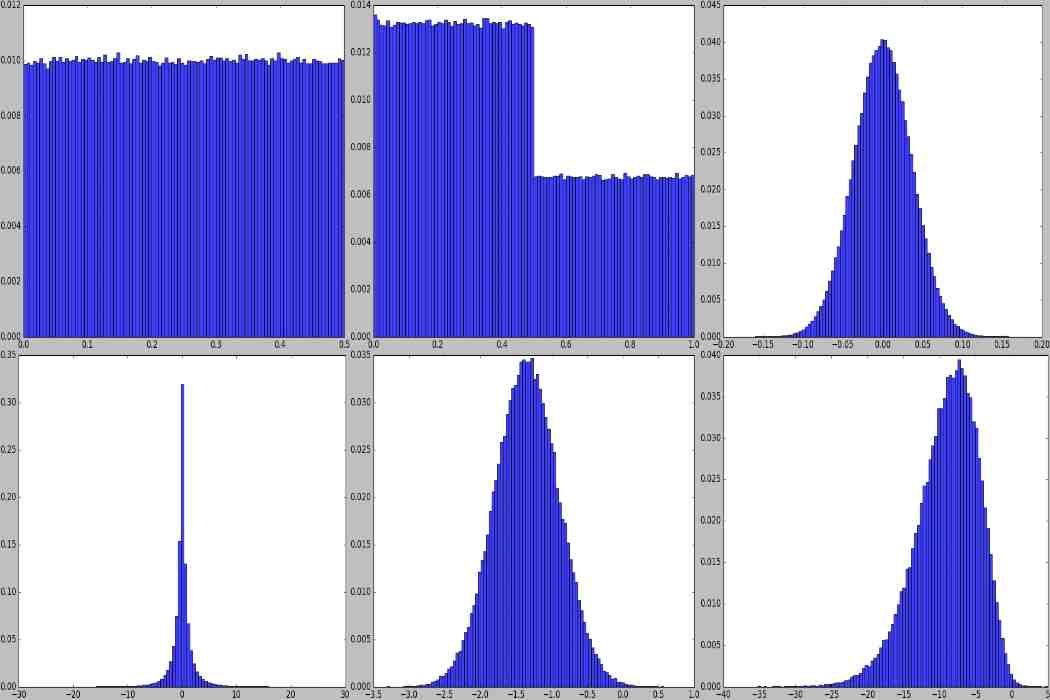• Power(param, val, elementwise): Same as Add, but raises sampled values to the exponent val. The shortcut is **. Example:

```from imgaug import parameters as iap
params = [
iap.Uniform(0, 1) ** 2, # identical to: Power(Uniform(0, 1), 2)
iap.Clip(iap.Uniform(-1, 1) ** iap.Normal(0, 1), -4, 4)
]
iap.show_distributions_grid(params, rows=1)
```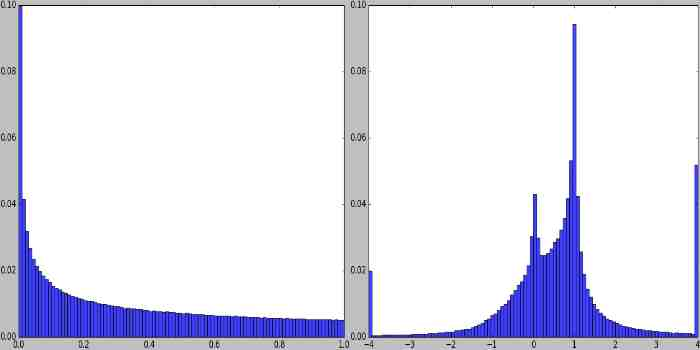## Special Parameters¶

• Deterministic(v): A constant. Upon sampling, this always returns v.

• Choice(values, replace=True, p=None): Upon sampling, this parameter picks randomly elements from a list values. If replace is set to True (default), the picking happens with replacement. By default, all elements have the same probability of being picked. This can be modified using p. Note that values may also contain strings and other stochastic parameters. In the latter case, each picked parameter will be replaced by a sample from that parameter. This allows merging of probability mass functions, but is a rather slow process. All elements in values should have the same datatype (except for stochastic parameters). Example:

```from imgaug import parameters as iap
params = [
iap.Choice([0, 1, 2]),
iap.Choice([0, 1, 2], p=[0.15, 0.5, 0.35]),
iap.Choice([iap.Normal(-3, 1), iap.Normal(3, 1)]),
iap.Choice([iap.Normal(-3, 1), iap.Poisson(3)])
]
iap.show_distributions_grid(params)
```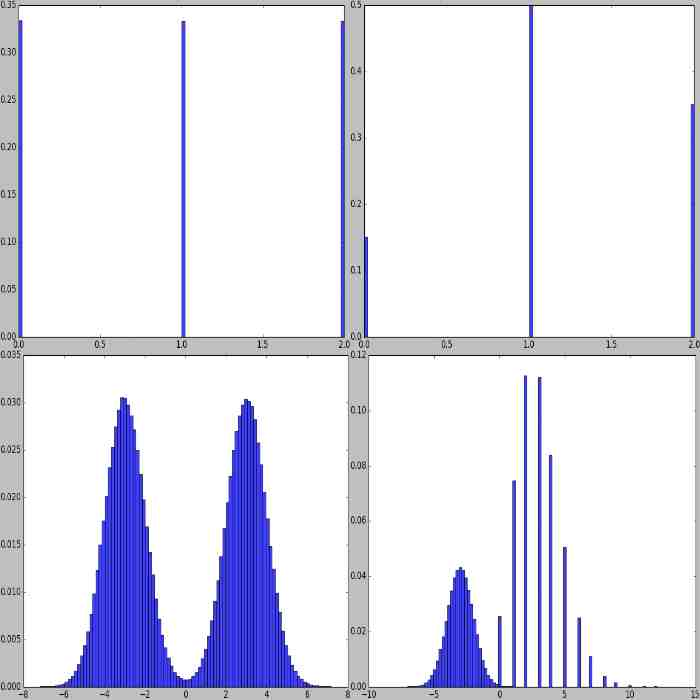• Clip(param, minval=None, maxval=None): Clips the values sampled from param to the range [minval, maxval]. minval and maxval may be None. In that case, only minimum or maximum clipping is applied (depending on what is None). Example:

```from imgaug import parameters as iap
params = [
iap.Clip(iap.Normal(0, 1), -2, 2),
iap.Clip(iap.Normal(0, 1), -2, None)
]
iap.show_distributions_grid(params, rows=1)
```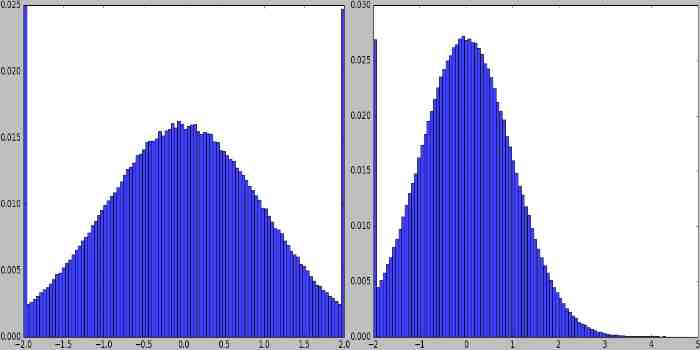• Discretize(param): Converts a continuous parameter param into a discrete one (using rounding). Discrete parameters are not changed. Example:

```from imgaug import parameters as iap
params = [
iap.Discretize(iap.Normal(0, 1)),
iap.Discretize(iap.ChiSquare(3))
]
iap.show_distributions_grid(params, rows=1)
```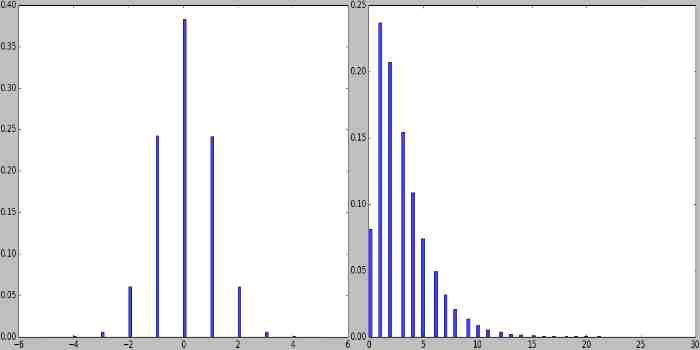• Absolute(param): Applies an absolute function to each value sampled from param, turning them to positive ones. Example:

```from imgaug import parameters as iap
params = [
iap.Absolute(iap.Normal(0, 1)),
iap.Absolute(iap.Laplace(0, 1))
]
iap.show_distributions_grid(params, rows=1)
```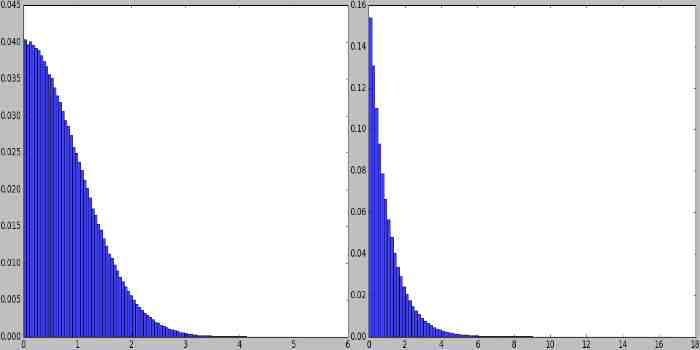• RandomSign(param, p_positive=0.5): Randomly flips the signs of values sampled from param. Optionally, the probability of flipping a value’s sign towards positive can be set. Example:

```from imgaug import parameters as iap
params = [
iap.ChiSquare(3),
iap.RandomSign(iap.ChiSquare(3)),
iap.RandomSign(iap.ChiSquare(3), p_positive=0.75),
iap.RandomSign(iap.ChiSquare(3), p_positive=0.9)
]
iap.show_distributions_grid(params)
```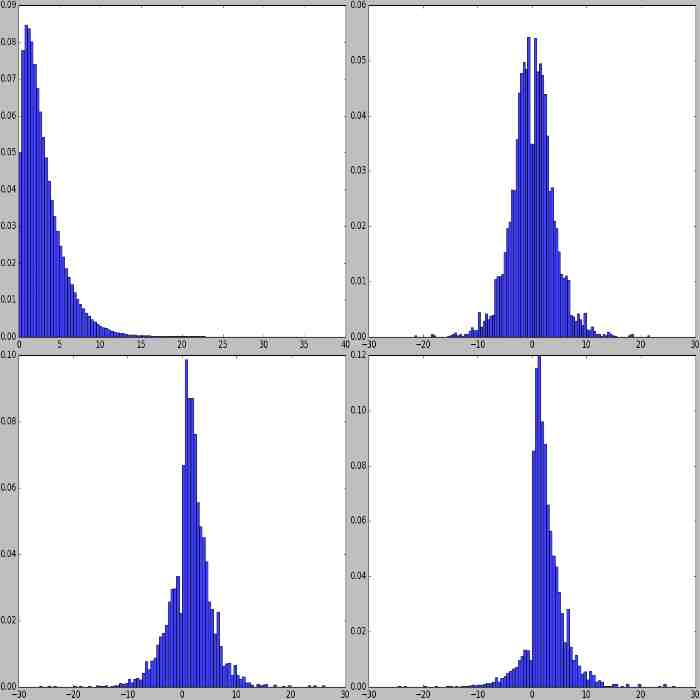• ForceSign(param, positive, mode=”invert”, reroll_count_max=2): Converts all values sampled from param to positive or negative ones. Signs of positive/negative values may simply be flipped (mode=”invert”) or resampled from param (mode=”reroll”). When rerolling, the number of iterations is limited to reroll_count_max (afterwards mode=”invert” is used). Example:

```from imgaug import parameters as iap
params = [
iap.ForceSign(iap.Normal(0, 1), positive=True),
iap.ChiSquare(3) - 3.0,
iap.ForceSign(iap.ChiSquare(3) - 3.0, positive=True, mode="invert"),
iap.ForceSign(iap.ChiSquare(3) - 3.0, positive=True, mode="reroll")
]
iap.show_distributions_grid(params)
```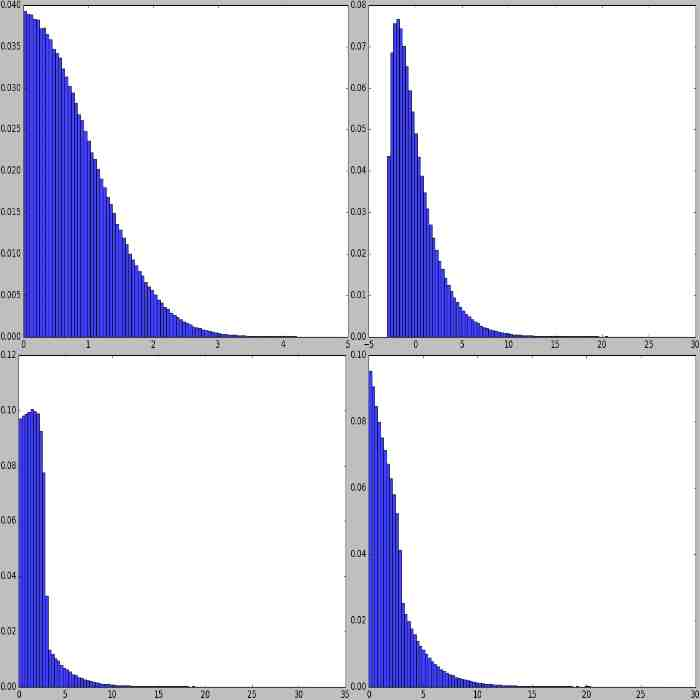• Positive(other_param, mode=”invert”, reroll_count_max=2): Shortcut for ForceSign with positive=True. E.g. Positive(Normal(0, 1)) restricts a normal distribution to only positive values.

• Negative(other_param, mode=”invert”, reroll_count_max=2): Shortcut for ForceSign with positive=False. E.g. Negative(Normal(0, 1)) restricts a normal distribution to only negative values.

• FromLowerResolution(other_param, size_percent=None, size_px=None, method=”nearest”, min_size=1): Intended for 2d-sampling processes, e.g. for masks. Samples these in a lower resolution space. E.g. instead of sampling a mask at 100x100, this allows to sample it at 10x10 and then upsample to 100x100. One advantage is, that this can be faster. Another possible use is, that the upsampling may result in large, correlated blobs (linear interpolation) or rectangles (nearest neighbour interpolation).

TODO